# Geometry lesson in plan

## Steiner's Theorem

Let's have the triangle ABC and the points M, N ∈(BC). Ifthen this relation is true: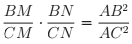## Proof.

We use small geometric constructions. We build BE || AC, E ∈AM and CF || AB,  F  ∈ AN.(angles with parallel sides) (1)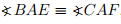(we know this from the hypothesys) (2)

From  (1) and (2) we have,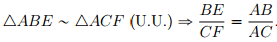BE || AC => (acc. Fundamental Theorem of Similarity)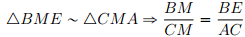CF || AB => (acc. Fundamental Theorem of Similarity)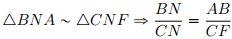From the last two relations we get: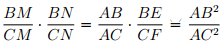Keywords: plan geometry theorems, theorem Steiner

### Forum

Here you can discuss about mathematic, about algebra, geometry, trigonometry.

It is not mandatory to be logged in on this forum but it is nice to have an account. You can ask about mathematics just with your name and your email.

This maths forum is one of the easiest forums to use it.

>> Go to Math Forum